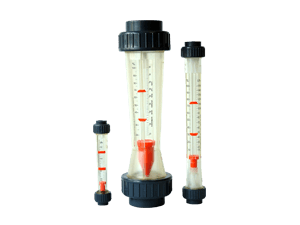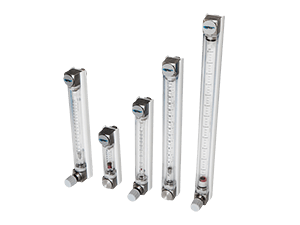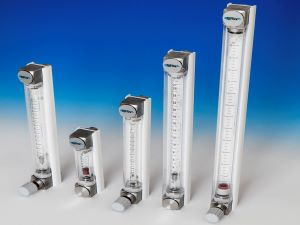# Variable Area Flow MetersDFM Variable Area Low cost Flow MeterNFX Glass Tube Low cost Flow MeterUniflux Flow Meters Variable Area Flow Meter

# Variable Area Flow Meters

The variable area flow meter’s operation is based on the variable area principle: fluid flow raises a float in a tapered tube, increasing the area for passage of the fluid. The greater the flow, the higher the float is raised.

The height of the float is directly proportional to the flowrate. With liquids, the float is raised by a combination of the buoyancy of the liquid and the velocity head of the fluid. With gases, buoyancy is negligible, and the float responds to the velocity head alone.

The float moves up or down in the tube in proportion to the fluid flow rate and the annular area between the float and the tube wall. The float reaches a stable position in the tube when the upward force exerted by the flowing fluid equals the downward gravitational force exerted by the weight of the float.

A change in flowrate upsets this balance of forces. The float then moves up or down, changing the annular area until it again reaches a position where the forces are in equilibrium. To satisfy the force equation, the variable area flow meter float assumes a distinct position for every constant flowrate.

However, it is important to note that because the float position is gravity dependent, variable area meters must be vertically oriented and mounted.

Flowquip offer a wide range of Variable Area flow meters for simple, cost effective measurement of liquids and gases. Call us today to discuss your application 01422 829920 or email sales@flowquip.co.uk